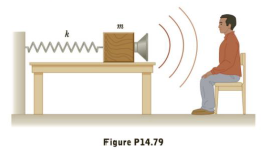Chapter 14, Problem 79AP

Chapter
Section
Textbook Problem

A block with a speaker bolted to it is connected to a spring having spring constant k = 20.0 N/m, as shown in Figure P14.79. The total mass of the block and speaker is 5.00 kg, and the amplitude of the unit’s motion is 0.500 m. If the speaker emits sound waves of frequency 440. Hz, determine the (a) lowest and (b) highest frequencies heard by the person to the right of the speaker.(a)

To determine
The lowest frequencies heard by the person to the right of the speaker.

Explanation

Given Info: The spring constant is k=20.0N/m , amplitude of the unit’s motion is 0.500 m, and mass of the block and speaker is 5.00 kg.

Formula to calculate the maximum velocity of the block and speaker, Apply principle of conservation of energy is,

KE=PE

• KE is the kinetic energy of the block and spring system.
• PE is the elastic potential energy of the spring system.

Use 1/2mvmax2 for KE and 1/2kA2 for PE .

12mvmax2=12kA2mvmax2=kA2vmax=kA2m

• k is the spring constant.
• A is the amplitude.
• m is the mass of the block and speaker.
• vmax is the maximum velocity of the block and speaker.

Substitute 20.0N/m , for k , 0.500 m for A, and 5.00 kg for m .

vmax=(20.0N/m)(0

(b)

To determine
The highest frequencies heard by the person to the right of the speaker.

Still sussing out bartleby?

Check out a sample textbook solution.

See a sample solution

The Solution to Your Study Problems

Bartleby provides explanations to thousands of textbook problems written by our experts, many with advanced degrees!

Get Started

Why does a stars life expectancy depend on its mass?

Horizons: Exploring the Universe (MindTap Course List)

Describe the general structure of a neuron.

Chemistry for Today: General, Organic, and Biochemistry

How do a solute, a solvent, and a solution differ?

Biology: The Dynamic Science (MindTap Course List)